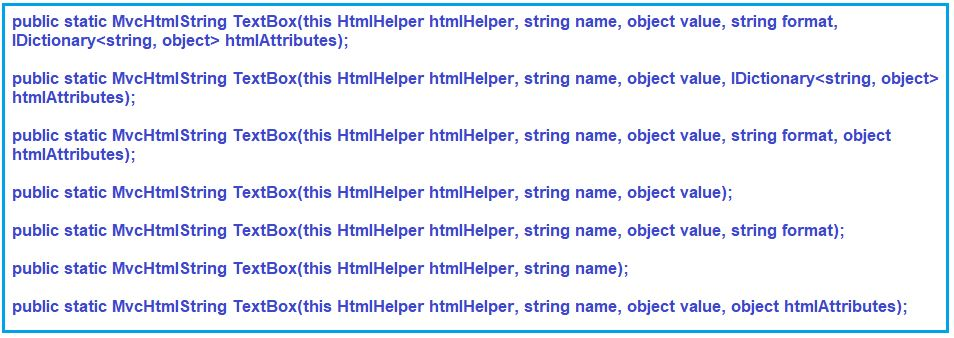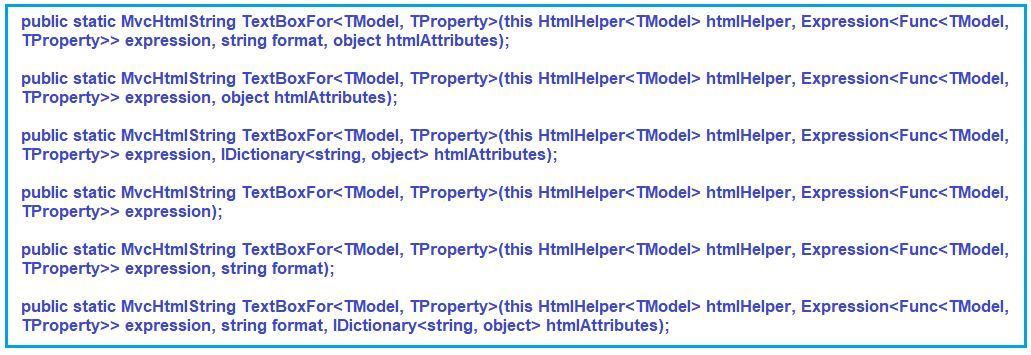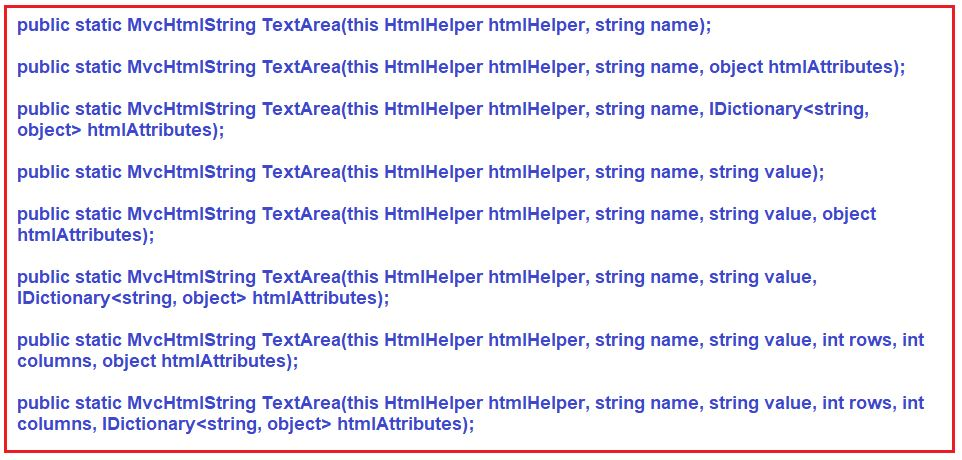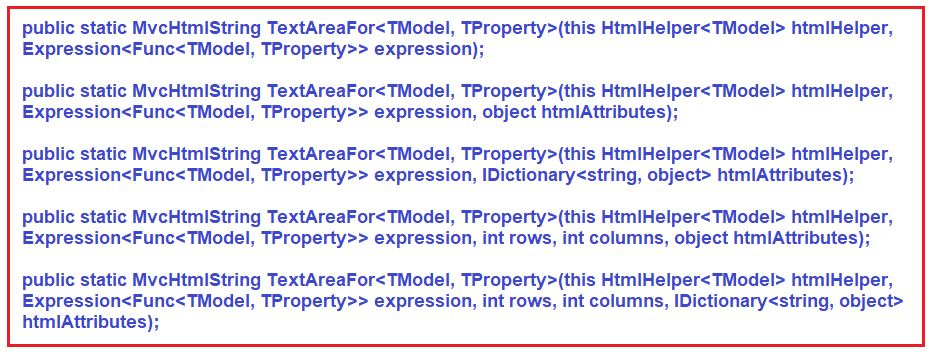# TextBox HTML Helper in ASP.NET MVC

## TextBox HTML Helper in ASP.NET MVC Application

In this article, I am going to discuss TextBox HTML Helper in ASP.NET MVC Application and along the way, we will also discuss how to create TextArea using HTML Helper in ASP.NET MVC application. Please read our previous article before proceeding to this article where we discussed the basis of HTML Helpers in the ASP.NET MVC Application. As part of this article, we are going to discuss the following pointers.

1. How to Create TextBox using HTML Helper in MVC?
2. TextBox() HTML Helper Method in ASP.NET MVC.
3. TextBoxFor() HTML Helper Method in ASP.NET MVC application
4. What are the Differences between Html.TextBox and Html.TextBoxFor in MVC Application?
5. How to Create TexArea using HTML Helper in MVC?
6. Understanding TextArea() and TextAreaFor() HTML Helper Method in MVC.
##### How to Create TextBox using HTML Helper in MVC?

To create a TextBox using HTML Helper Method in the ASP.NET MVC application, we need to use the TextBox Helper method. In the ASP.NET MVC application, we can use two different types of TextBox Helper methods to generates a textbox in a view. Those two extension methods are TextBox() and TextBoxFor(). The TextBox() HTML Helper method is a loosely typed method whereas the TextBoxFor() HTML Helper method is a strongly typed method.

##### Let’s understand How to Create TextBox using HTML Helper in MVC with one example:

Let’s create an ASP.NET MVC 5 application with the name HTML_HELPER. Then Create Employee.cs class file within the Models folder and then copy and paste the following code into it.

```using System.ComponentModel.DataAnnotations;
namespace HTML_HELPER.Models
{
public class Employee
{
public int EmployeeId { get; set; }
[Display(Name = "Name")]
public string EmployeeName { get; set; }
public string Password { get; set; }
public string Gender { get; set; }
public string City { get; set; }
public Nullable<decimal> Salary { get; set; }
}
}
```

We are going to use the above Employee model with TextBox() and TextBoxFor() HTML Helper methods. Once you create the Employee model next we are going to create an MVC 5 Empty Controller with the name EmployeeController within the Controllers Folder.

```namespace HTML_HELPER.Controllers
{
public class EmployeeController : Controller
{
public ActionResult Index()
{
return View();
}
}
}
```

The EmployeeController is created with one action method i.e. the Index action method. So, create the respective Index view with empty HTML.

##### TextBox() HTML Helper Method in ASP.NET MVC:

The Html.TextBox() Helper method creates an element of <input type=”text”> with specified name, value and HTML attributes. There 7 overloaded versions of this Html.TextBox() Helper method is available as shown in the below image. The following method are loosely typed method###### Parameters:
1. htmlHelper: The HTML helper instance that this method extends. It indicates that it is an extension method that belongs to HtmlHelper class.
2. name: The name of the form field and the System.Web.Mvc.ViewDataDictionary key that is used to look up the value.
3. value: The value of the text input element. The value is retrieved in this order – the System.Web.Mvc.ModelStateDictionary object, the value of this parameter, the System.Web.Mvc.ViewDataDictionary object, and lastly, a value attribute in the HTML attributes.
4. format: A string that is used to format the input.
5. htmlAttributes: An object that contains the HTML attributes to set for the element.

Returns: This method returns an input element whose type attribute is set to “text”.

Note: Replace Home with Employee in RouteConfig class.

##### Modify the Index View:

Please modify the Index View as shown below to use the TextBox Helper method.
@model HTML_HELPER.Models.Employee
@Html.TextBox(“EmployeeName”, null, new { @class = “form-control” })

Run the application and inspect the Html and you will see that it will produce the following HTML for the textbox.
<input class=”form-control” id=”EmployeeName” name=”EmployeeName” type=”text” value=””>

In the above example, the first parameter is “EmployeeName” which is a property of the Employee model which will be set as the name and id of the textbox. The second parameter is the value that we need to display in the textbox, which is null in the above example because the TextBox() method will automatically display the value of the EmployeeName property of the Employee model. The third parameter will be set as the class attribute. The HTML attributes parameter is an object type, so it can be an anonymous object, and the attributes name will be its properties starting with @ symbol.

You can also specify any name for the textbox. However, it will not be bind to a model.
@Html.TextBox(“myTextBox”, “This is value”, new { @class = “form-control” })

It will produce the following HTML
<input class=”form-control” id=”myTextBox” name=”myTextBox” type=”text” value=”This is value”>

##### TextBoxFor() HTML Helper Method in ASP.NET MVC application:

The TextBoxFor() HTML Helper method is a strongly typed extension method. It generates an element of <input type=”text”> for the model property which needs to be specified using a lambda expression. The TextBoxFor() HTML Helper method binds the specified model object property to the input element. So it automatically displays that model property value within the textbox. The TextBoxFor() HTML Helper Method has 6 overloaded versions available in ASP.NET MVC Framework are shown in the below image.###### Parameters:
1. htmlHelper: The HTML helper instance that this method extends.
2. expression: An expression that identifies the object that contains the properties to display.
3. format: A string that is used to format the input.
4. htmlAttributes: An object that contains the HTML attributes to set for the element.

Type parameters:

1. TModel: The type of model.
2. TProperty: The type of the value.

Returns: An input element whose type attribute is set to “text”.

##### Example to understand the TextBoxFor() HTML Helper Method in MVC:

Copy and Paste the following code in Index.cshtml view
@model HTML_HELPER.Models.Employee
@Html.TextBoxFor(m => m.EmployeeName, new { @class = “form-control” })

Run the application and insepct the element and you will see that, it will produce the following Html
<input class=”form-control” id=”EmployeeName” name=”EmployeeName” type=”text” value=””>

In the above example, the first parameter in TextBoxFor() HTML Helper Method is a lambda expression which specifies the EmployeeName property of the Model object to bind with the textbox. It generates an input type text element with id and name property and both the properties value are set to EmployeeName. The value attribute will be set to the value of the EmployeeName property of the Model Object.

##### What are the Differences between Html.TextBox and Html.TextBoxFor in ASP.NET MVC application?

As we already discussed that the @Html.TextBox() is a loosely typed helper method whereas the @Html.TextBoxFor() is a strongly typed helper method.

The Html.TextBox() Helper method is not strongly typed and hence they don’t require a strongly typed view. This means that we can hardcode whatever name we want. On the other hand the Html.TextBoxFor() HTML Helper method is a strongly typed method and hence it requires a strongly typed view and the name should be given using the lambda expression. What is a strongly typed view that we will discuss in our upcoming articles?

The Strongly typed HTML helper methods also provide compile-time error checking. In real-time applications, we mostly prefer to use strongly typed HTML Helper methods.

But the most important point that we need to keep in mind is whether we use Html.TextBox Helper method or Html.TextBoxFor() Helper method the end result is the same that is they generate the same HTML.

Note: The Strongly typed HTML helpers are introduced in MVC2.

##### How to Create TexArea using HTML Helper in ASP.NET MVC Application?

To create TextArea using HTML Helper in ASP.NET MVC application, we need to use the TextArea Helper methods.  The HtmlHelper class provides two extension methods to generate the textarea i.e. multiline textbox in a razor view: They are TextArea() and TextAreaFor(). By default, the textarea is created with rows=2 and cols=20 size.

##### TextArea() HTML Helper Method in MVC:

The Html.TextArea() method creates an input HTML element of <textarea rows=”2″ cols=”20″> with a specified name, value and HTML attributes. The TextArea() HTML Helper method is a loosely typed helper method because the name parameter is a string. The name parameter can also be the name of a property of a model object. It binds the specified property with the textarea. As a result, it automatically displays that property value within the textarea. There are also 8 overloaded versions available for TextArea() HTML Helper Method in ASP.NET MVC Framework as shown in the below image.###### Parameters:
1. htmlHelper: The HTML helper instance that this method extends.
2. name: The name of the form field to return.
3. value: The text content.
4. rows: The number of rows.
5. columns: The number of columns.
6. htmlAttributes: An object that contains the HTML attributes to set for the element.

Returns: Returns the specified textarea element by using the specified HTML helper, the name of the form field, the text content, the number of rows and columns, and the specified HTML attributes.

##### Example: TextArea HTML Helper Method in ASP.NET MVC

Let’s modify our Employee Model as shown below

```using System.ComponentModel.DataAnnotations;
namespace HTML_HELPER.Models
{
public class Employee
{
public int EmployeeId { get; set; }
[Display(Name = "Name")]
public string EmployeeName { get; set; }
public string Password { get; set; }
public string Gender { get; set; }
public string City { get; set; }
public Nullable<decimal> Salary { get; set; }
public string Address { get; set; }
}
}
```
###### Modify the Employee Controller as shown below:
```namespace HTML_HELPER.Controllers
{
public class EmployeeController : Controller
{
public ActionResult Index()
{
Employee emp = new Employee()
{
EmployeeId = 1,
Address = "Andheri, Sakinaka, Mumbai, 400097, Maharashtra, India"
};
return View(emp);
}
}
}
```
###### Modify the Index View:

Copy and Paste the following code in the Index view
@model HTML_HELPER.Models.Employee
@Html.TextArea(“Address”, null, new { @class = “form-control” })

If you inspect the text area, then you will see that it will produce the following HTML

In the above example, the first parameter is the “Address” property of the Employee model class which will be set as name and id of the textarea. The second parameter is the value to display in the textarea, which is null in the above example because TextArea() method will automatically display a value of the Address property in the textarea. The third parameter will be set as a class attribute. The HtmlAttributes parameter is an object type, so it can be an anonymous object, and attributes name will be its properties starting with @ symbol.

We can also specify any name for the textarea. However, it will not be bound to a model.
@Html.TextArea(“myTextArea”, “This is value”, new { @class = “form-control” })

It will produce the following html
<textarea class=”form-control” cols=”20″ id=”myTextArea” name=”myTextArea” rows=”2″>This is value</textarea>

##### TextAreaFor() HTML Helper Method in ASP.NET MVC:

The TextAreaFor() HTML Helper method is a strongly typed extension method. It generates a text area input element. We need to specify the name using a lambda expression. The TextAreaFor() HTML Helper Method binds that specified model object property to the textarea element. So it automatically displays that model property value within the textarea. There are also 5 overloaded versions available for TextAreaFor() HTML Helper Method in ASP.NET MVC Framework as shown in the below image.###### Parameters:
1. htmlHelper: The HTML helper instance that this method extends.
2. expression: An expression that identifies the object that contains the properties to render.
3. rows: The number of rows.
4. columns: The number of columns.
5. htmlAttributes: A dictionary that contains the HTML attributes to set for the element.

Type parameters:

1. TModel: The type of model.
2. TProperty: The type of property.

Returns: Returns an HTML textarea element for each property in the object that is represented by the specified expression using the specified HTML attributes and the number of rows and columns.

##### Example: TextAreaFor HTML Helper Method in ASP.NET MVC:

Copy and paste the following code in the index view
@model HTML_HELPER.Models.Employee
@Html.TextAreaFor(m => m.Address, new { @class = “form-control” })

It will produce the following HTML